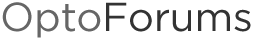Modbus Inputs PAC Control

Does anyone know how to bring Modbus data into PAC Project in four digit binary groups, BCD? The data coming in reads 584 which is the decimal value of binary 10 0100 1000. But, that is not what I need. I need it to read it in groups of 4 starting with the LSB. So, it would be 248, which represents 24.8 degrees C.

There are a couple ways to do this. Since the BCD representation is equivalent to the hexadecimal representation, you can use a couple built in functions:

NumberToHexString(BCD, HexString);
Decimal = StringToInt32(HexString);

I think it is a bit of a hack, and probably low performance, but it works.

A mathematical way to do this with masking and bit shifting is likely faster:

Decimal = (BCD bitand 0x00F)
+ ((BCD bitand 0x0F0) >> 4) * 10
+ ((BCD bitand 0x0F00) >> 8) * 100
+ ((BCD bitand 0x0F000) >> 12) * 1000;

At this point you can divide the result by 10.0 and store in a float to get the scaled value.

2 Likes

Thank you so much. It worked perfectly.